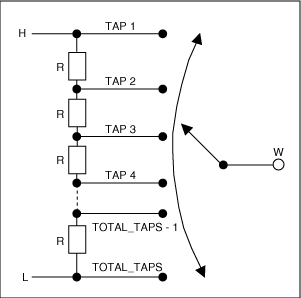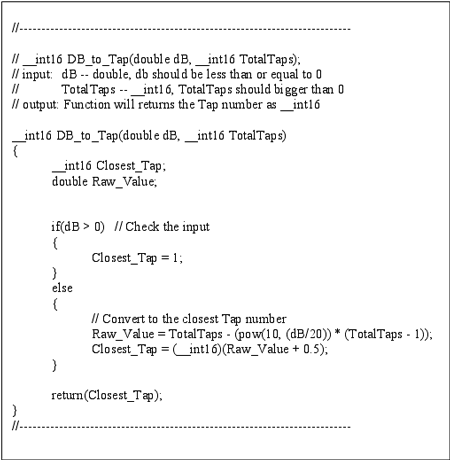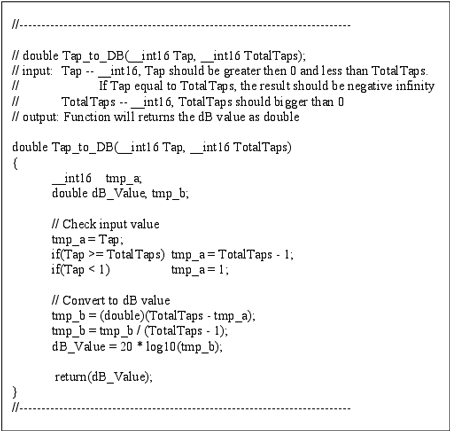# Logging the Linear Digital Potentiometer

### Abstract

Digital potentiometers (digital pots or digipots) are commonly used in volume-control applications. For audio applications, volume attenuation should closely mimic human hearing by applying a logarithmic scale. In some designs, a simple, low-cost linear potentiometer can be used in place of a logarithmic-taper potentiometer (log pot). This application note details how to implement a linear potentiometer in a log-taper application using a small, low-cost, higher resolution digipot.

### Introduction

Because of their resiliency, small size, and ease of use, digital potentiometers have become widely accepted as replacements for mechanical potentiometers, particularly in volume-control applications. In this type of audio application, it is often preferable to use a log-taper pot instead of a linear-taper pot because the human ear perceives the logarithmic attenuation of sound as linear attenuation. However, as this application note demonstrates, a high-resolution linear potentiometer can also be used to implement volume control. The following analysis shows how to use a linear digital pot as a log pot (Figure 1).Figure 1. This family of digital pots is configured in a standard manner, as they include a connection to both the high end and the low end of the resistor string, as well as a wiper connection that moves among the resistor string's tap points.

### Using a Linear Digipot as a Log Pot

Using simple math and a basic understanding of linear-taper digital potentiometers, it is possible to realize a logarithmic taper from a linear-taper pot. Because a potentiometer is essentially a voltage-divider, its output voltage (VOUT) can be written in terms of the voltage applied to its input VIN, if VIN is applied at RH. The following equation can be used to express this relationship:

VOUT = VIN(RW-L / RH-L)(Eq. 1)

where RW-L is the resistance between the wiper (W) and the low end of the resistor string (L) and RH-L is the total end-to-end resistance of the resistor string.

The attenuation of a given signal in decibels can be found using the following equation:

Attenuation (dB) = 20 * log(VOUT / VIN)(Eq. 2)

Substituting the VOUT value from Equation 1 into Equation 2 yields the following equation:

Attenuation (dB) = 20 * log[VIN(RW-L / RH-L)/VIN] = 20 * log(RW-L / RH-L)(Eq. 3)

Figure 2 shows that these resistances can be represented by the tap position (RW-L) and the total number of taps (RH-L).Figure 2. Linear-potentiometer tap points are located at equal resistor segments along the entire resistor string.

The tap position (RW-L) can therefore be represented as:

RW-L = (Total_Taps - x) * R(Eq. 4)

where x = 1, 2, 3...Total_Taps.

Because the first tap point (minimum attenuation) contains no resistance from the resistor string, the total end-to-end resistance can be represented as RH-L = (Total_Taps - 1) * R. Now, the attenuation equation takes the form of:

Attenuation (dB) = 20 * log[(Total_Taps - x) / (Total_Taps - 1)](Eq. 5)

where xTotal_Taps. If x = Total_Taps, then the attenuation approaches -.

### Conclusion

The above procedure details how to convert a linear-taper digital potentiometer into a logarithmic-taper potentiometer. This conversion works best with higher resolution linear pots (128 taps or greater) because logarithmic resolution is severely limited for linear pots with fewer than 128 tap points. Also, some inaccuracies may be encountered at the endpoints due to other internal structures, such as ESD protection or the nonlinear resistance of a pass transistor being used as a switch. The following source code converts desired attenuation to tap position (Figure 3), as well as desired tap position to the corresponding attenuation (Figure 4).Figure 3. This source code converts desired attenuation to tap position.Figure 4. This source code converts desired tap position to the corresponding attenuation.

A similar article appeared in the April 4, 2005 edition of EE Times.